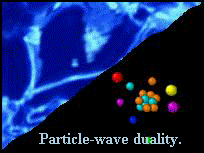﻿ Home
Quantum Mechanics
Introduction

Quantum mechanics is a mathematical theory that can describe the behavior of objects that are roughly 10,000,000,000 times smaller than a typical human being. Quantum particles move from one point to another as if they are waves. However, at a detector they always appear as discrete lumps of matter. There is no counterpart to this behavior in the world that we perceive with our own senses. One cannot rely on every-day experience to form some kind of "intuition" of how these objects move.The intuition or "understanding" formed by the study of basic elements of quantum mechanics is essential to grasp the behavior of more complicated quantum systems.

The approach adopted in all textbooks on quantum mechanics is that the mathematical solution of model problems brings insight in the physics of quantum phenomena. The mathematical prerequisites to work through these model problems are considerable. Moreover, only a few of them can actually be solved analytically. Furthermore, the mathematical structure of the solution is often complicated and presents an additional obstacle for building intuition.

This presentation introduces the basic concepts and fundamental phenomena of quantum physics through a combination of computer simulation and animation. The primary tool for presenting the simulation results is computer animation. Watching a quantum system evolve in time is a very effective method to get acquainted with the basic features and peculiarities of quantum mechanics. The images used to produce the computer animated movies shown in this presentation are not created by hand but are obtained by visualization of the simulation data. The process of generating the simulation data for the movies requires the use of computers that are far more powerful than Pentium III based PC´s. At the time that these simulations were carried out (1994), most of them required the use of a supercomputer. Consequently, within this presentation, it is not possible to change the model parameters and repeat a simulation in real time.

This presentation is intended for all those who are interested to learn about the fundamentals of quantum mechanics. Some knowledge of mathematics will help but is not required to understand the basics. This presentation is not a substitute for a textbook. The presentation begins by showing the simplest examples, such as the motion of a free particle, a particle in an electric field, etc.. Then, the examples become more sophisticated in the sense that one can no longer rely on one´s familiarity with classical physics to describe some of the qualitative features seen in the animations. Classical notions are of no use at all for the last set of examples. However, once all other examples have been "understood", it should be possible to "explain" the behavior of these systems also. Instead of using a comprehensive mathematical apparatus to obtain and analyze solutions of model problems, a computer simulation technique is employed to solve these problems including those that would prove intractable otherwise.